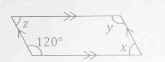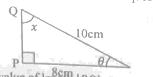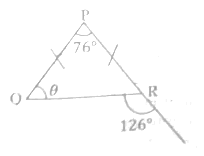# NDA 2015 MATHEMATICS Past Questions and Answers

Scroll Down and click on link or Go to Link for destination

# NDA MATHEMATICS 2015 PAST QUESTIONS AND ANSWERS1. The area of a square is 144 sq cm. Find the length of the diagonal.

A. 13 cm
B. 12√2 cm
C. 12 cm
D. 11√3cm

2. Find the number of ways of selecting 6 out of 10 subjects for an examination.
A. 218
B. 216
C. 215
D. 210

3. Find the principal which amount to ₦5,500 at simple interest in 5 years at 2% per annum.

A. ₦5,000
B. 4,900
B. ₦4,800
D. 4,700

4. The sixth term of an arimethic progression is half of its twelfth term. The first term is equal to __.
A. half of the common difference
B. double the common difference
C. the common difference
D. zero

5. Find the value of [(3.7)]2 – (15.3)2]1/2 leaving your answer correct to 4 significant figures.

A. 30.03
B. 35.00
C. 31.01
D. 30.12

6. If 126n = 86, find a positive value for n.
A. 2
B. 4
C. 8
D. 10A. x = 5 or x = -4
B. x = 3 or x = -5
C. x = 4 or x = -5
D. x = 0 or x = -2

8. A regular polygon is a polygon with:

A. Equal sides with different angles
B. Equal sides with equal angles
C. None of the above
D. All of the above

9. From the diagram below, find the value of xA. 1141/2˚
B. 1131/2˚
C. 1121/2˚
D. 1151/2˚

10. From the diagram below find the value of x, if |AO| = 5cm. and |BO| = 13cm to the nearest whole number.A. 12 cm
B.  8 cm
C.  10 cm
D.  14 cm

Use the diagram below to answer questions (11) and (12)11. Find the value of angle z
A. 200°
B. 160°
C. 60°
D. 80°

12. Find the value of angle y.
A. 140°
B. 120°
C. 130°
D. 110°

13. Determine the basic solutions of 2cos2 Ɵ + cos Ɵ — 1 = 0
A. ᴨ
B. 2ᴨ
C. /3
D. nᴨ

Use the given figure below to answer question (14)14. What is the value of length |PQ|
A. 6.5 cm
B. 6 cm
C. 5.5 cm
D. 6.2 cm

15. Find the median of 6,5,9,8,4,7,2,8,9,4
A. 6
B. 6.5
C. 7
D. 7.5

16. Ogive is defined as

A. Smooth curve obtained by plotting cumulative frequency against upper class
boundary
B. Smooth curve obtained by plotting cumulative frequency against lower class boundary
C. Smooth curve obtained by plotting cumulative frequency against the mean.
D. None of the above

17. Find the distance between the points A(-1, 4) and B(-2, -3).
A. 25√2
B. 5√2
C. 50√2
D. 50

18. Evaluate |AC| the triangle with vertex A(-1, 2), B(1, -3) and C(3,2)
A. 16
B. 13
D. 41/2
D. 17

19. Find the area of triangle ABC with vertices A(2, 1), B(2, 4) and C(-2, 1)

A. 6
B. 4
C. 8
D. 5

20.  Find where the straight line 3x + 4y = 7 intercepts the coordinate axis.21. Find the equation of the line with gradient -1/3 and passing through (2, -1)
A. x - 3y - 1 = 0
B. x - 3y + 1 = 0
C. X + 3y + 1 = 0
D. x- 3y + 2 =0

22. Find equation of the line through (2,3) and is perpendicular to the line 2x -3y +2=0
A.2y – 3x - 12 = 0
B. 2y + 3x - 12 = 0
C. 2y + 3x + 12 = 0
D. 3x - 2y + 12 = 0
A. 0.01285
B. 0.1286
C. 0.1285
D. 0.01286

24. A trader bought goats for ₦4000 each and sold all of them for ₦180,000 at a loss 25%. How many goats did he buy?

A. 50
B. 60
C. 36
D. 45

25. If Musa scored 75 in Biology instead of 57, average mark in four subjects would have been 60. What was his total mark?
A. 282
B. 240
C. 222
D. 210

26. Divide the L.C.M of 48, 64, and 80 by their H. C. F

A. 20
B. 30
C. 48
D. 60
27. In the diagram above, what is the perimeter of the equilateral triangle ABC
A. 3
B. 9
C. 10
D. 30

28. A pie chart of total number of students in a school has a sector 35° representing 14 students. How many students are there in the school together?

A. 200
B. 140
C. 144
D. 250

29. If a block of height 2.5 cm represents 100 goats, how high should the block be to represent 170 goats.
A. 4.50 cm
B. 5.00 cm
C. 4.00 cm
D. 4.25 cm

30. The sum of the 10th terms of an AP is 30 and its 12th term is 36. Find the first term and the common difference.

A. 19.77, 5.07
B. -19.77, 5.07
C. 19.77. -5.07
D -19.77, -5.07

31. If T varies inversely proportional to P and directly to U, formulate the problem
A. T = K/P+U
B. T α 1/P
C. T α LI
D. T = K/P + AU

32. Find the equation of the tangent to the curve y = x3 at the point (2, 8)
A. 12x + y — 16 = 0
B. 12x — 2y + 16 = 0
C. 12x — y — 16 = 0
D. 12x — y + 16 = 0

33. A hunter 1.6m tall, views a bird on top of a tree at an angle 45˚. If the distance between the hunter and the tree is 10.4m, find the height of the tree.

A. 12.0 m
B. 10.4 m
C. 9.0 m
D. 8.8 m

34. The sum of the interior angles of a polygon is 20 right angles. How many sides the polygon have?
A. 40
B. 20
C. 10
D. 1235. The triangle PQR above is
A. an isosceles triangle
B. an obtuse-angled triangle
C. a scalene triangle
D. an equilateral triangle

36 Find the standard deviation of the numbers 1, 7, 6, 3, 4, 5, 2, 8, 9
A. 6
B. √6
C. 7
D. √7

37. Find the arithmetic mean of the following numbers 30, 32, 34, 36, 38, 40, 42

A. 34
B. 38
C. 36
D. 37

38. Five years ago, a father was 3 times as old as his son. Now, their combined ages amount to 110 years. What is the current age of the father is?

A. 75 years
B. 60 years
C. 98 years
D. 80 years

39. Find the product of (2√y— 3y) and (3y + 2√y)
A. 4y + y2
B. 4y + 9y2
C. 4y - 9y2
D. -4y - 9y2

40. A number of pencils were shared out among Bisi, Sola and Tunde in the ratio of 2:3:5 respectively. If Bisi got 5, how many were shared out?

A. 15
B. 25
C. 30
D. 50